# NCERT Class VII: Science-Exercise Solutions

### Chapter 15-Light-Exercise Solutions

NCERT Book Page Number-189
Q.1. Fill in the blanks:
(a) An image that cannot be obtained on a screen is called ____________.
(b) Image formed by a convex __________ is always virtual and smaller in size.
(c) An image formed by a __________ mirror is always of the same size as that of the object.
(d) An image which can be obtained on a screen is called a _________ image.
(e) An image formed by a concave ___________ cannot be obtained on a screen.
Answer:
(a) An image that cannot be obtained on a screen is called virtual image.
(b) Image formed by a convex mirror is always virtual and smaller in size.
(c) An image formed by a plane mirror is always of the same size as that of the object.
(d) An image which can be obtained on a screen is called a real image.
(e) An image formed by a concave lens cannot be obtained on a screen.

Q.2. Mark ‘T’ if the statement is true and ‘F’ if it is false:
(a) We can obtain an enlarged and erect image by a convex mirror. (T/F)
(b) A concave lens always forms a virtual image. (T/F)
(c) We can obtain a real, enlarged and inverted image by a concave mirror. (T/F)
(d) A real image cannot be obtained on a screen. (T/F)
(e) A concave mirror always forms a real image. (T/F)
Answer:
(a) We can obtain an enlarged and erect image by a convex mirror. (F)
Explanation: –
Image formed by a convex mirror is always diminished and erect.
(b) A concave lens always forms a virtual image. (T)
(c) We can obtain a real, enlarged and inverted image by a concave mirror. (T)
(d) A real image cannot be obtained on a screen. (F)
Explanation: –
A real image is always obtained on a screen.
(e) A concave mirror always forms a real image. (F)
Explanation: –
Concave mirrors can form both real and virtual images.
Q.3. Match the columns: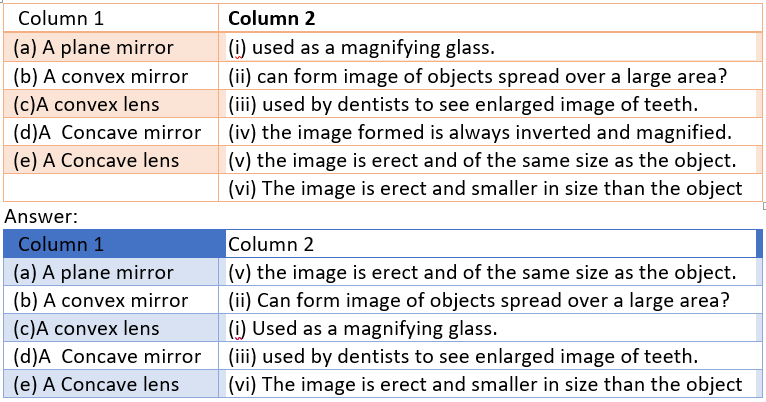Q.4. State the characteristics of the image formed by a plane mirror.
Answer: Image formed by a plane mirror has the following characteristics:
• Virtual and erect.
• Behind the mirror.
• Size of image is equal to size of object.
• Laterally inverted image (image of left side visible on right side).
• Distance of image behind the mirror is same as distance of object from mirror.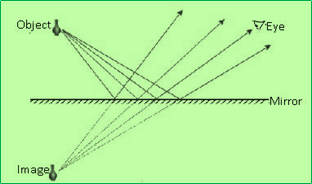Q.5. Find out the letters of English alphabet or any other language known to you in which the image formed in a plane mirror appears exactly like the letter itself. Discuss your findings?
Answer: A, H, I, M, O, T, U, V, W and X are the letters which form same image as the letter is. These letters are laterally symmetrical.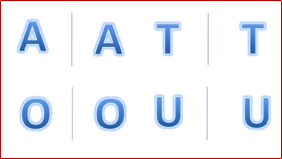NCERT Book Page Number-190
Q.6. What is a virtual image? Give one situation where a virtual image is formed?
Answer: A virtual image is formed when reflected rays appear to meet.
• Such images cannot be obtained on screen.
• Plane mirrors, convex mirror and concave lens always forms virtual image.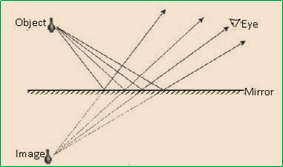Q.7. State two differences between convex and a concave lens?
Answer: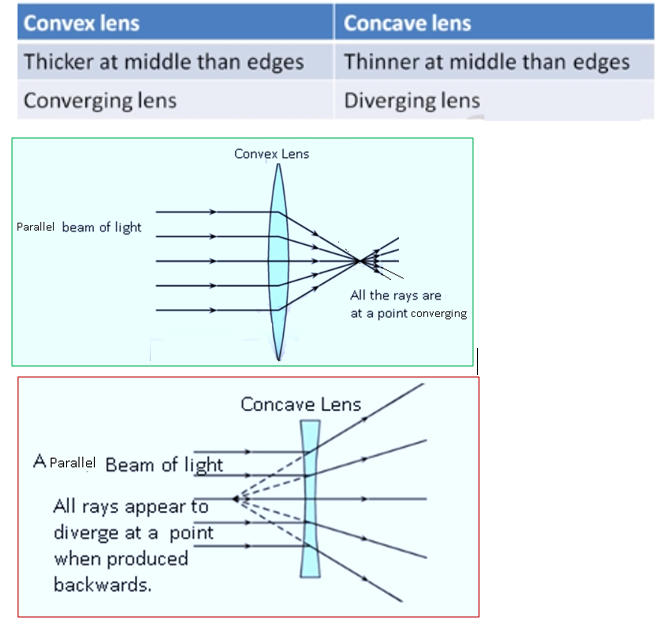Q.8. Give one use each of a concave and a convex mirror.
Answer:
Uses of Concave and Convex mirror are following:-
• Concave mirror is used by dentist, solar furnace, reflector of a torch, etc.
• Convex mirror is used in rear view mirrors.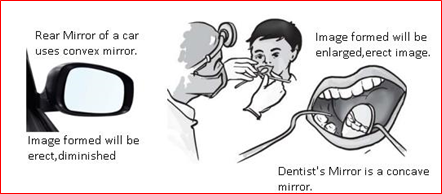Q.9. Which type of mirror can form a real image?
Answer: Concave mirrors can form real images. Convex mirrors always forms virtual image.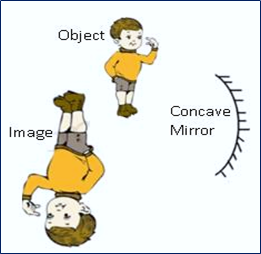Q.10. Which type of lens forms always a virtual image?
Answer: Concave lens will always form virtual, erect and diminished images.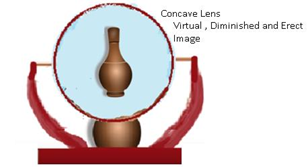Choose the correct option in questions 11–13
Q.11. A virtual image larger than the object can be produced by a
(i) concave lens
(ii) concave mirror
(iii) convex mirror
(iv) plane mirror
Answer: Correct option is (ii).
Q.12. A virtual image larger than the object can be produced by a concave mirror.
David is observing his image in a plane mirror. The distance between the mirror and his image is 4 m. If he moves 1 m towards the mirror, then the distance between David and his image will be
(i) 3 m
(ii) 5 m
(iii) 6 m
(iv) 8 m
Answer: Correct option is (iii) 6m.
Initially David is standing in front of the mirror.
The distance between him and his image= 4m.
David moves 1m towards the mirror.
The distance of David from the mirror will be 3m.
Distance of the image from the mirror = 3m.
Therefore the distance between David and his image= 3m + 3m =6m.
Q.13. The rear view mirror of a car is a plane mirror. A driver is reversing his car at a speed of 2 m/s. The driver sees in his rear view mirror the image of a truck parked behind his car. The speed at which the image of the truck appears to approach the driver will be
(i) 1 m/s
(ii) 2 m/s
(iii) 4 m/s
(iv) 8 m/s
Answer: Correct option is (ii) 2m/s.
As the car moves 2m backward, the mirror also moves 2m backward, so image comes 2 m forward.